Learn all Concepts of Chapter 4 Class 10 (with VIDEOS). Check - Quadratic Equations - Class 10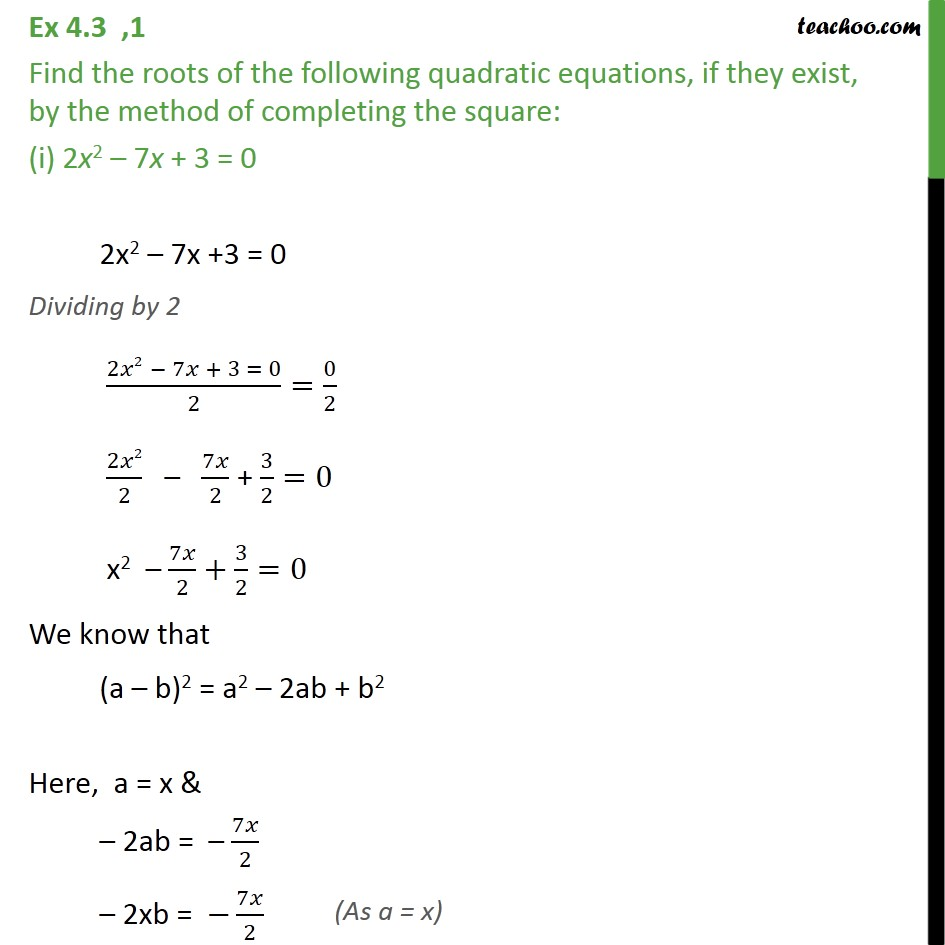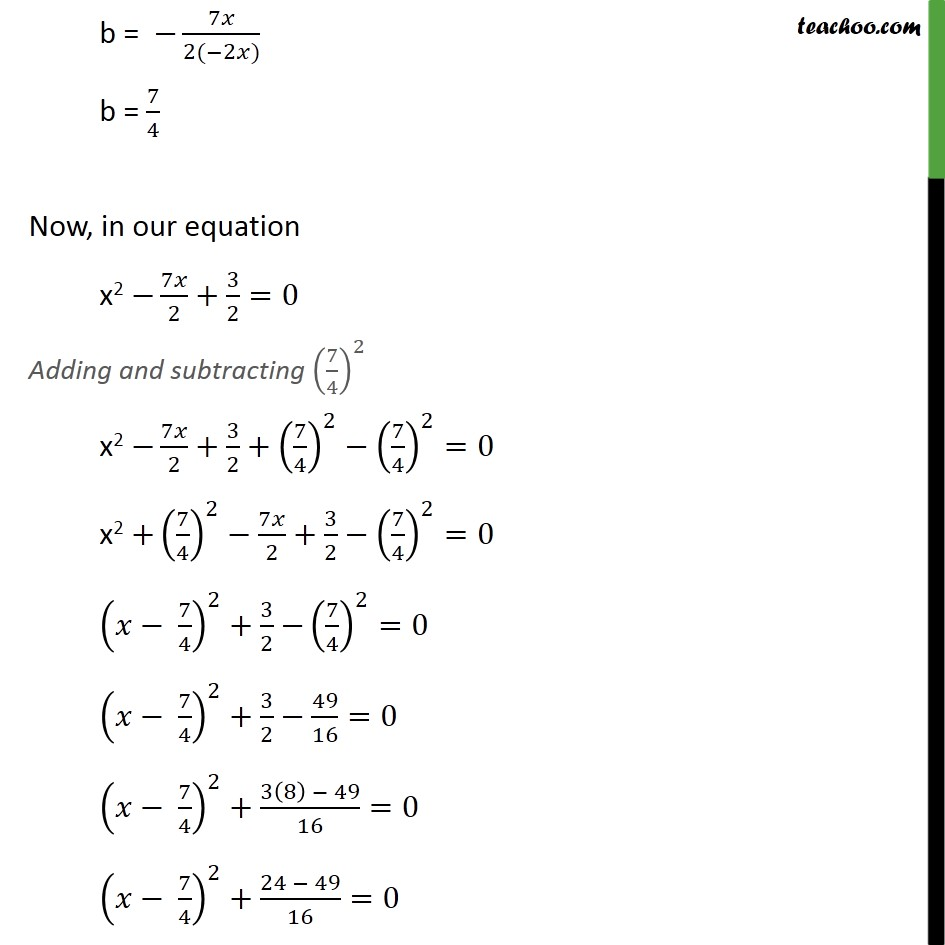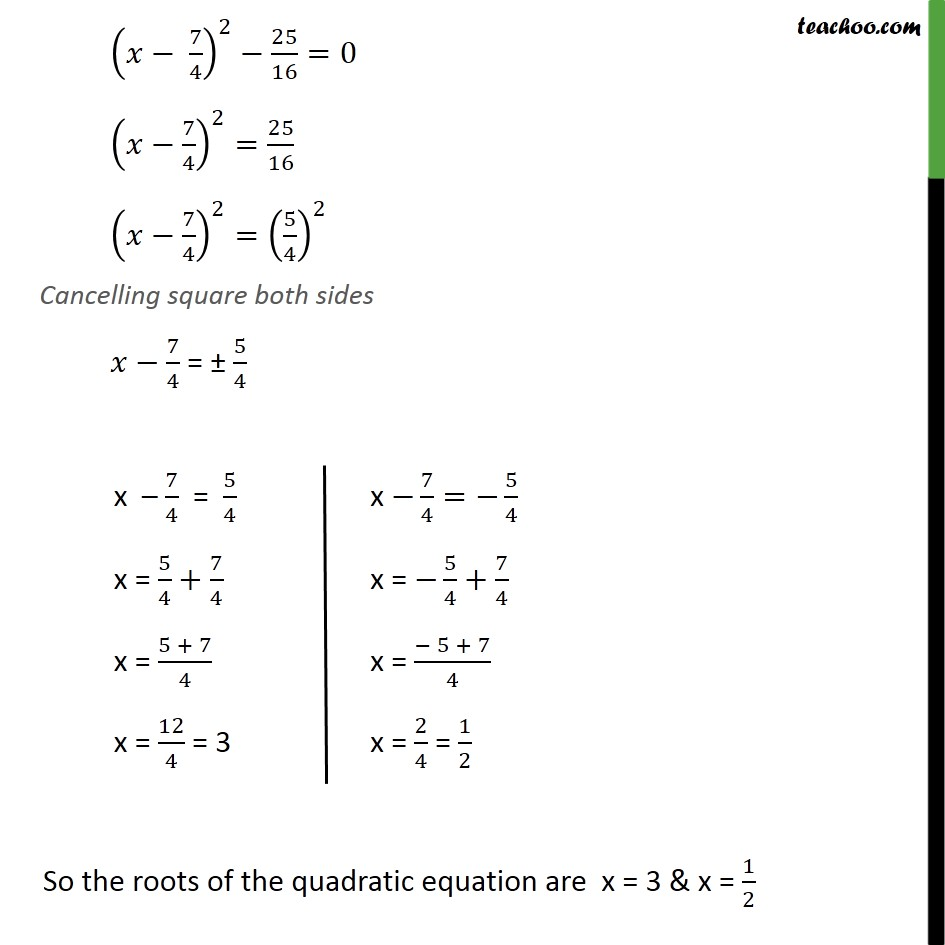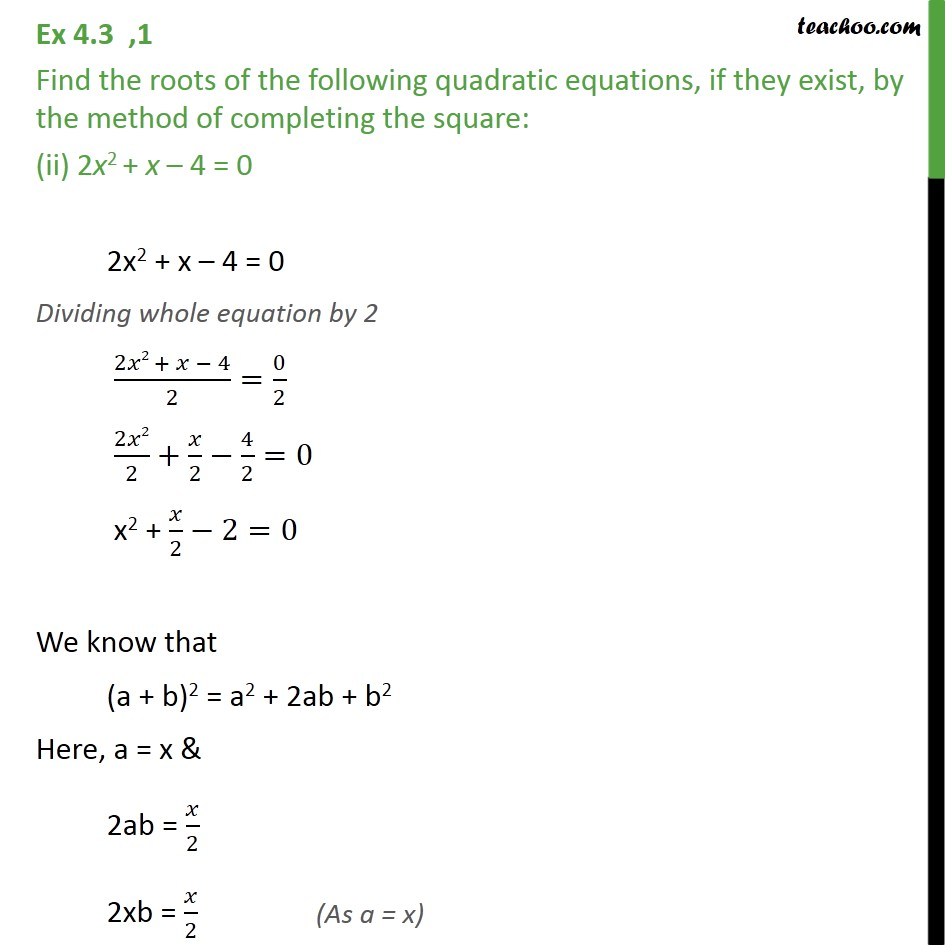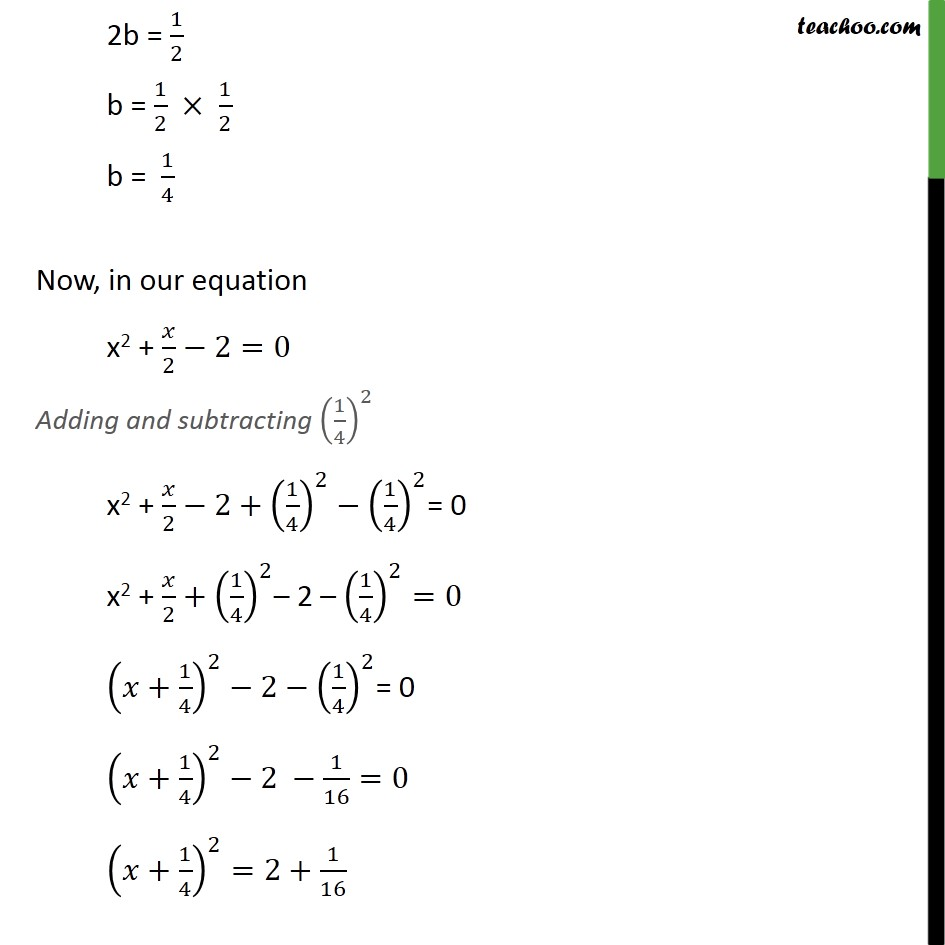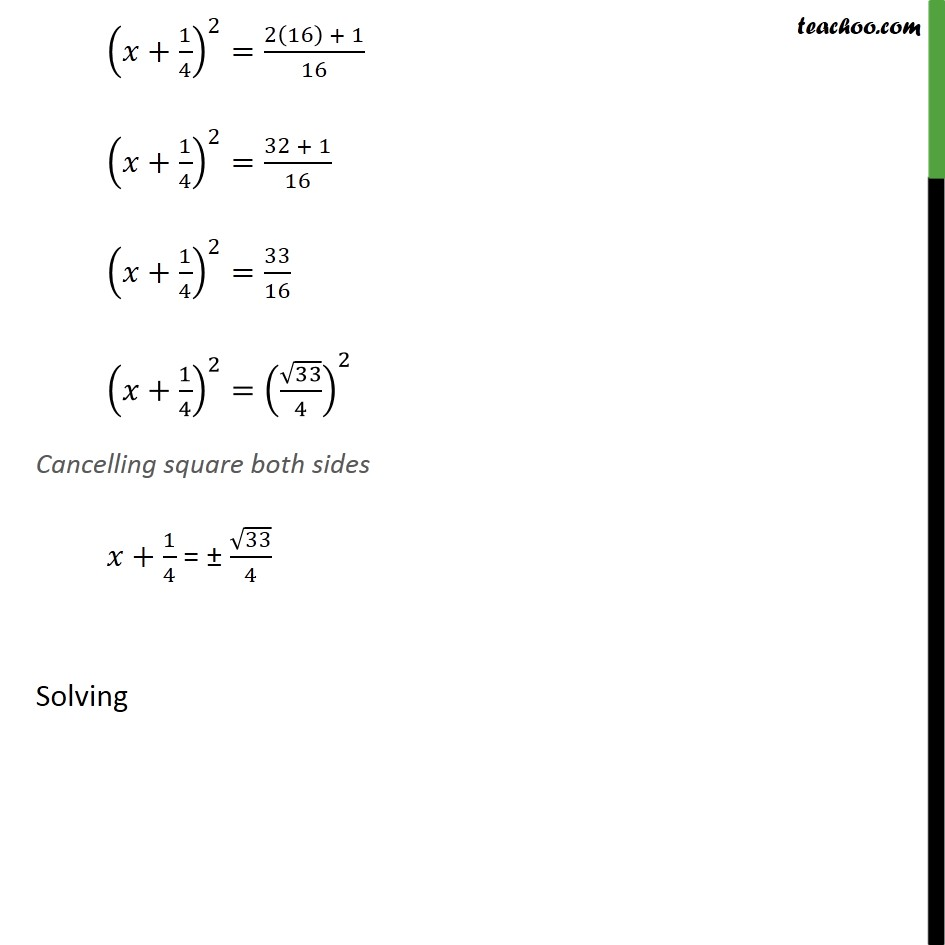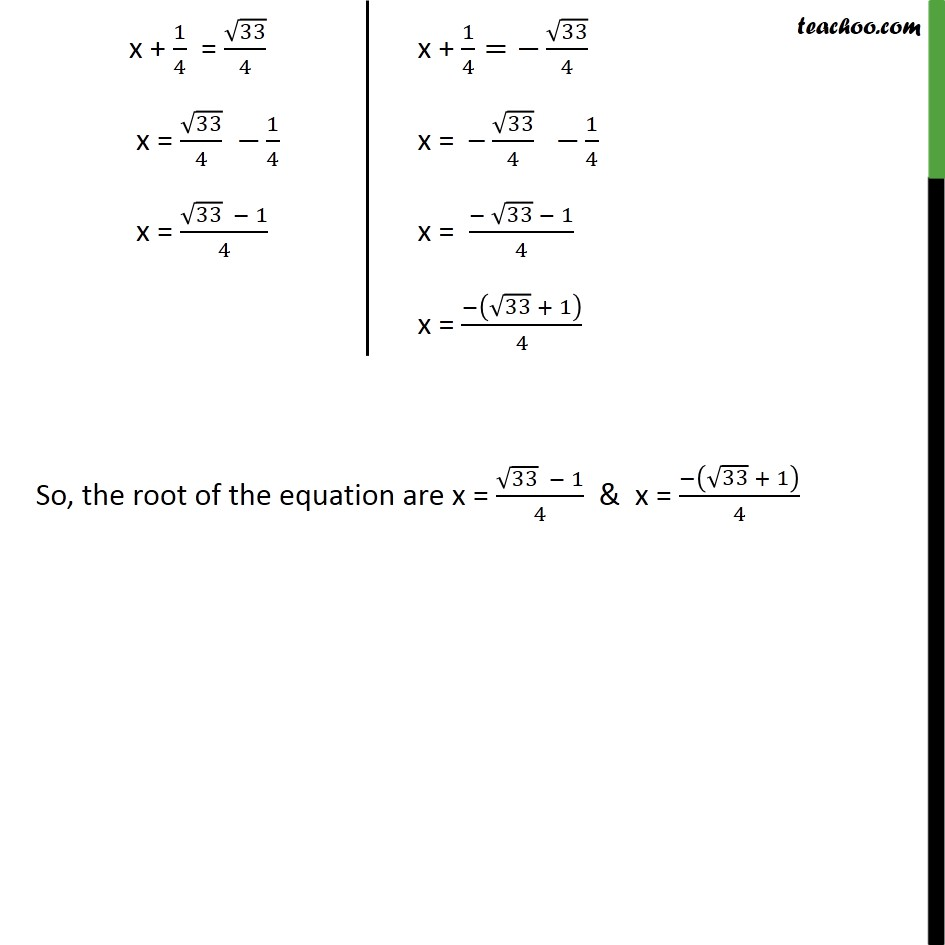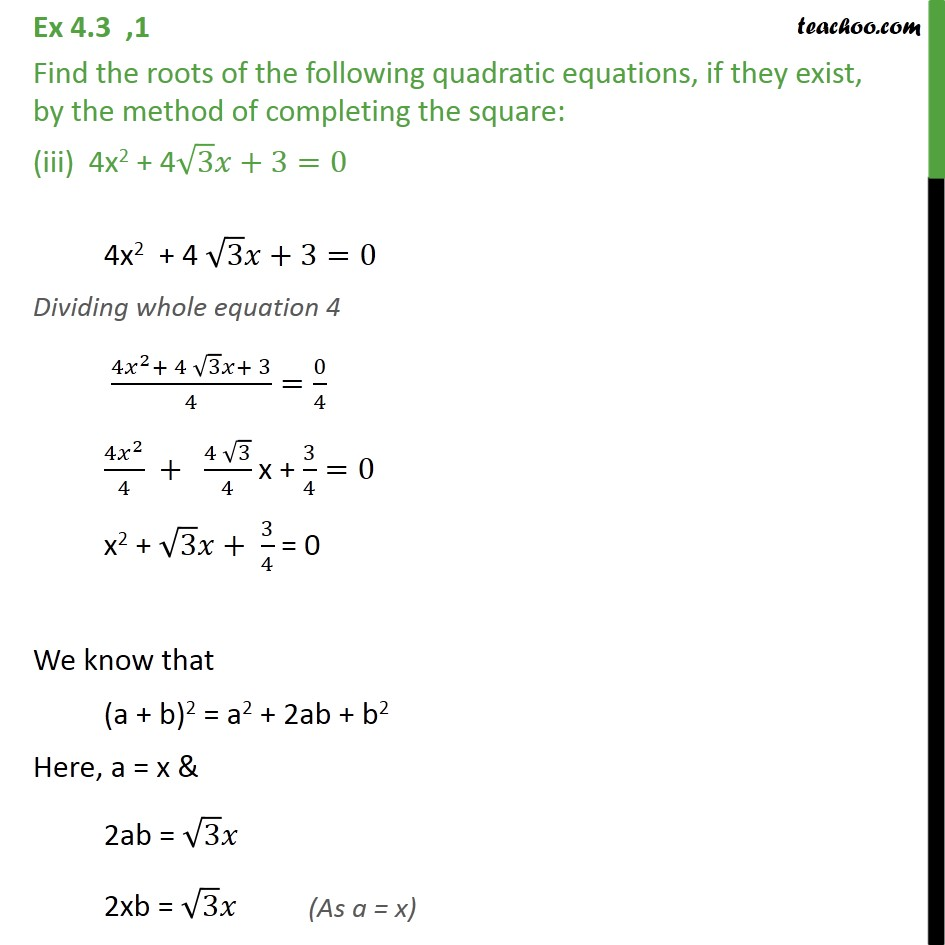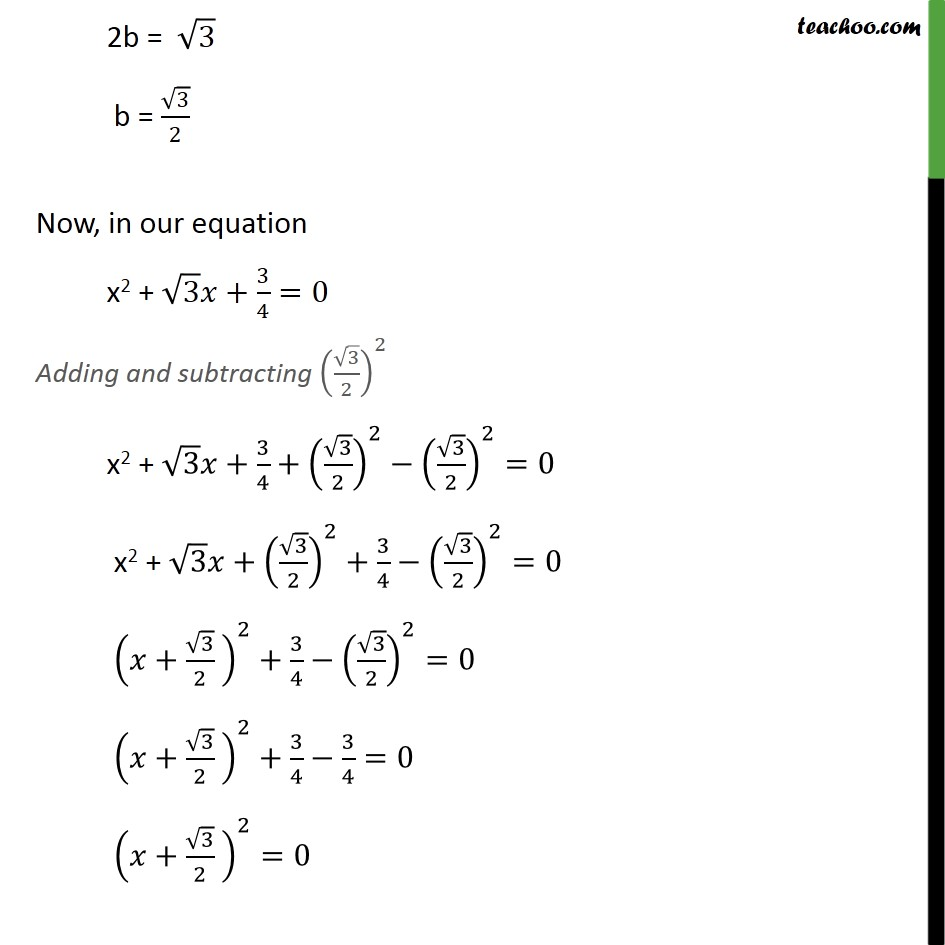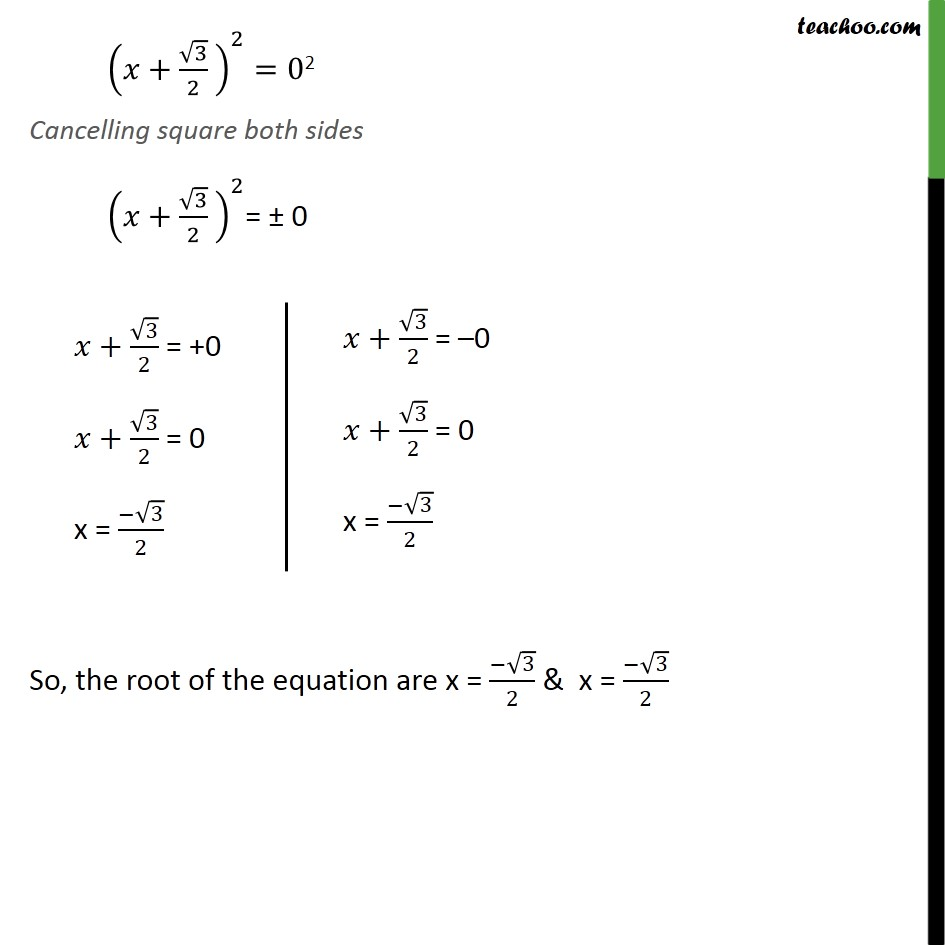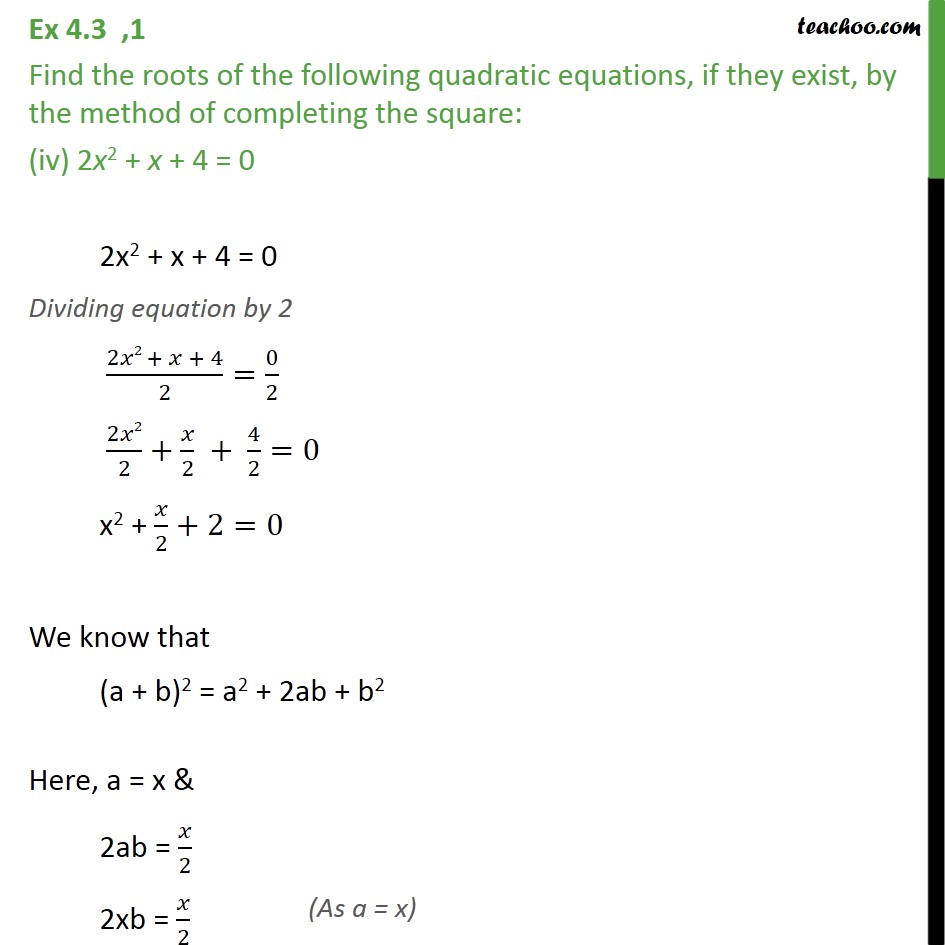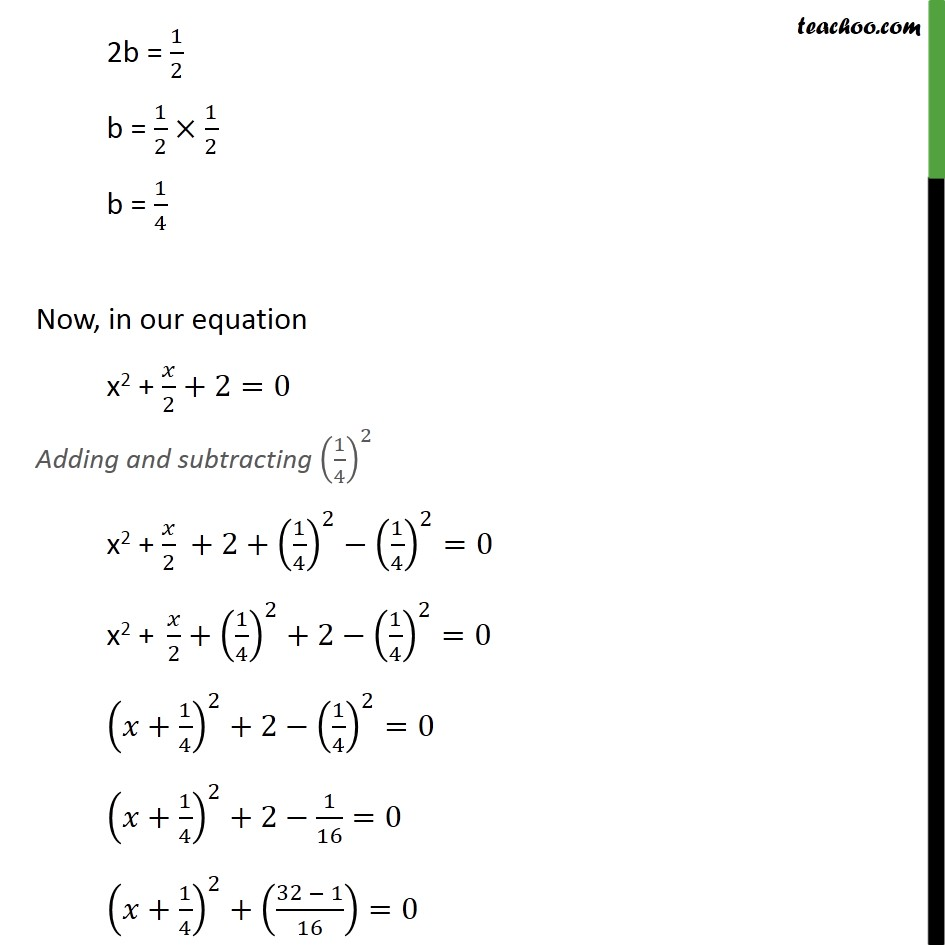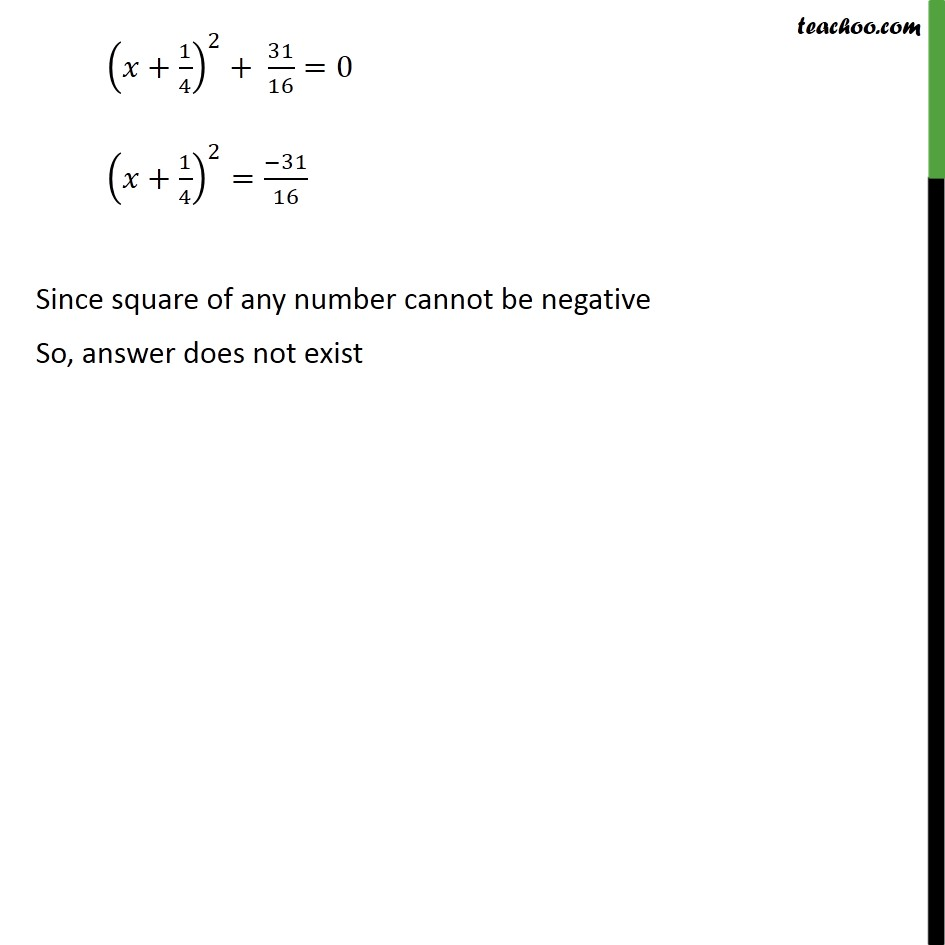1. Chapter 4 Class 10 Quadratic Equations
2. Serial order wise
3. Ex 4.3

Transcript

Ex 4.3 ,1 Find the roots of the following quadratic equations, if they exist, by the method of completing the square: (i) 2x2 – 7x + 3 = 0 2x2 – 7x +3 = 0 Dividing by 2 (2𝑥2 − 7𝑥 + 3 = 0)/2=0/2 2𝑥2/2 – 7𝑥/2 + 3/2=0 x2 – 7𝑥/2+3/2=0 We know that (a – b)2 = a2 – 2ab + b2 Here, a = x & – 2ab = – 7𝑥/2 – 2xb = −7𝑥/2 b = −7𝑥/(2(−2𝑥)) b = 7/4 Now, in our equation x2 −7𝑥/2+3/2=0 Adding and subtracting (7/4)^2 x2 −7𝑥/2+3/2+(7/4)^2−(7/4)^2=0 x2 +(7/4)^2−7𝑥/2+3/2−(7/4)^2=0 (𝑥− 7/4)^2+3/2 −(7/4)^2=0 (𝑥− 7/4)^2+3/2−49/16=0 (𝑥− 7/4)^2+(3(8) − 49)/16=0 (𝑥− 7/4)^2+(24 − 49)/16=0 (𝑥− 7/4)^2−25/16=0 (𝑥−7/4)^2=25/16 (𝑥−7/4)^2=(5/4)^2 Cancelling square both sides 𝑥−7/4 = ± 5/4 Ex 4.3 ,1 Find the roots of the following quadratic equations, if they exist, by the method of completing the square: (ii) 2x2 + x – 4 = 0 2x2 + x – 4 = 0 Dividing whole equation by 2 (2𝑥2 + 𝑥 − 4)/2=0/2 2𝑥2/2+𝑥/2−4/2=0 x2 + 𝑥/2−2=0 We know that (a + b)2 = a2 + 2ab + b2 Here, a = x & 2ab = 𝑥/2 2xb = 𝑥/2 2b = 1/2 b = 1/2 × 1/2 b = 1/4 Now, in our equation x2 + 𝑥/2−2=0 Adding and subtracting (1/4)^2 x2 + 𝑥/2−2+(1/4)^2−(1/4)^2= 0 x2 + 𝑥/2+(1/4)^2– 2 – (1/4)^2=0 (𝑥+1/4)^2−2−(1/4)^2= 0 (𝑥+1/4)^2−2 −1/16=0 (𝑥+1/4)^2=2+1/16 (𝑥+1/4)^2=(2(16) + 1)/16 (𝑥+1/4)^2=(32 + 1)/16 (𝑥+1/4)^2=33/16 (𝑥+1/4)^2=(√33/4)^2 Cancelling square both sides 𝑥+1/4 = ± √33/4 Solving So, the root of the equation are x = (√33 − 1)/4 & x = (−(√33 + 1))/4 Ex 4.3 ,1 Find the roots of the following quadratic equations, if they exist, by the method of completing the square: (iii) 4x2 + 4√3 𝑥+3=0 4x2 + 4 √3 𝑥+3=0 Dividing whole equation 4 (4𝑥^2+ 4 √3 𝑥+ 3)/4=0/4 (4𝑥^2)/4 + (4 √3)/4 x + 3/4=0 x2 + √3 𝑥+ 3/4 = 0 We know that (a + b)2 = a2 + 2ab + b2 Here, a = x & 2ab = √3 𝑥 2xb = √3 𝑥 2b = √3 b = √3/2 Now, in our equation x2 + √3 𝑥+3/4=0 Adding and subtracting (√3/2)^2 x2 + √3 𝑥+3/4+(√3/2)^2−(√3/2)^2=0 x2 + √3 𝑥+(√3/2)^2+3/4−(√3/2)^2=0 (𝑥+√3/2 )^2+3/4−(√3/2)^2=0 (𝑥+√3/2 )^2+3/4−3/4=0 (𝑥+√3/2 )^2=0 (𝑥+√3/2 )^2=02 Cancelling square both sides (𝑥+√3/2 )^2= ± 0 So, the root of the equation are x = (−√3)/2 & x = (−√3)/2 Ex 4.3 ,1 Find the roots of the following quadratic equations, if they exist, by the method of completing the square: (iv) 2x2 + x + 4 = 0 2x2 + x + 4 = 0 Dividing equation by 2 (2𝑥2 + 𝑥 + 4)/2=0/2 2𝑥2/2+𝑥/2 + 4/2=0 x2 + 𝑥/2+2=0 We know that (a + b)2 = a2 + 2ab + b2 Here, a = x & 2ab = 𝑥/2 2xb = 𝑥/2 2b = 1/2 b = 1/2×1/2 b = 1/4 Now, in our equation x2 + 𝑥/2+2=0 Adding and subtracting (1/4)^2 x2 + 𝑥/2 +2+(1/4)^2−(1/4)^2=0 "x2 + " 𝑥/2+(1/4)^2+2−(1/4)^2=0 (𝑥+1/4)^2+2−(1/4)^2=0 (𝑥+1/4)^2+2−1/16=0 (𝑥+1/4)^2+((32 − 1)/16)=0 (𝑥+1/4)^2+ 31/16=0 (𝑥+1/4)^2=(−31)/16 Since square of any number cannot be negative So, answer does not exist

Ex 4.3